Documentation

### This is machine translation

Mouseover text to see original. Click the button below to return to the English version of the page.

To view all translated materials including this page, select Country from the country navigator on the bottom of this page.

## Maximizing Monochromatic Polarized Light Interference Patterns Using GlobalSearch and MultiStart

This example shows how to use the functions `GlobalSearch` and `MultiStart`.

### Introduction

This example shows how Global Optimization Toolbox functions, particularly `GlobalSearch` and `MultiStart`, can help locate the maximum of an electromagnetic interference pattern. For simplicity of modeling, the pattern arises from monochromatic polarized light spreading out from point sources.

The electric field due to source i measured in the direction of polarization at point x and time t is

``

whereis the phase at time zero for source,is the speed of light,is the frequency of the light,is the amplitude of source, andis the distance from sourceto.

For a fixed pointthe intensity of the light is the time average of the square of the net electric field. The net electric field is sum of the electric fields due to all sources. The time average depends only on the sizes and relative phases of the electric fields at. To calculate the net electric field, add up the individual contributions using the phasor method. For phasors, each source contributes a vector. The length of the vector is the amplitude divided by distance from the source, and the angle of the vector,is the phase at the point.

For this example, we define three point sources with the same frequency () and amplitude (), but varied initial phase (). We arrange these sources on a fixed plane.

```% Frequency is proportional to the number of peaks relFreqConst = 2*pi*2.5; amp = 2.2; phase = -[0; 0.54; 2.07]; numSources = 3; height = 3; % All point sources are aligned at [x_i,y_i,z] xcoords = [2.4112 0.2064 1.6787]; ycoords = [0.3957 0.3927 0.9877]; zcoords = height*ones(numSources,1); origins = [xcoords ycoords zcoords]; ```

### Visualize the Interference Pattern

Now let's visualize a slice of the interference pattern on the plane z = 0.

As you can see from the plot below, there are many peaks and valleys indicating constructive and destructive interference.

```% Pass additional parameters via an anonymous function: waveIntensity_x = @(x) waveIntensity(x,amp,phase, ... relFreqConst,numSources,origins); % Generate the grid [X,Y] = meshgrid(-4:0.035:4,-4:0.035:4); % Compute the intensity over the grid Z = arrayfun(@(x,y) waveIntensity_x([x y]),X,Y); % Plot the surface and the contours figure surf(X,Y,Z,'EdgeColor','none') xlabel('x') ylabel('y') zlabel('intensity') ```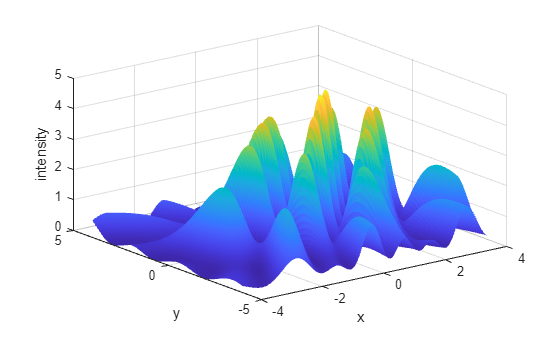### Posing the Optimization Problem

We are interested in the location where this wave intensity reaches its highest peak.

The wave intensity () falls off as we move away from the source proportional to. Therefore, let's restrict the space of viable solutions by adding constraints to the problem.

If we limit the exposure of the sources with an aperture, then we can expect the maximum to lie in the intersection of the projection of the apertures onto our observation plane. We model the effect of an aperture by restricting the search to a circular region centered at each source.

We also restrict the solution space by adding bounds to the problem. Although these bounds may be redundant (given the nonlinear constraints), they are useful since they restrict the range in which start points are generated (see the documentation for more information).

Now our problem has become:

``

subject to

``
``
``
``
``

whereandare the coordinates and aperture radius of thepoint source, respectively. Each source is given an aperture with radius 3. The given bounds encompass the feasible region.

The objective () and nonlinear constraint functions are defined in separate MATLAB® files, `waveIntensity.m` and `apertureConstraint.m`, respectively, which are listed at the end of this example.

### Visualization with Constraints

Now let's visualize the contours of our interference pattern with the nonlinear constraint boundaries superimposed. The feasible region is the interior of the intersection of the three circles (yellow, green, and blue). The bounds on the variables are indicated by the dashed-line box.

```% Visualize the contours of our interference surface domain = [-3 5.5 -4 5]; figure; ezcontour(@(X,Y) arrayfun(@(x,y) waveIntensity_x([x y]),X,Y),domain,150); hold on % Plot constraints g1 = @(x,y) (x-xcoords(1)).^2 + (y-ycoords(1)).^2 - 9; g2 = @(x,y) (x-xcoords(2)).^2 + (y-ycoords(2)).^2 - 9; g3 = @(x,y) (x-xcoords(3)).^2 + (y-ycoords(3)).^2 - 9; h1 = ezplot(g1,domain); h1.Color = [0.8 0.7 0.1]; % yellow h1.LineWidth = 1.5; h2 = ezplot(g2,domain); h2.Color = [0.3 0.7 0.5]; % green h2.LineWidth = 1.5; h3 = ezplot(g3,domain); h3.Color = [0.4 0.4 0.6]; % blue h3.LineWidth = 1.5; % Plot bounds lb = [-0.5 -2]; ub = [3.5 3]; line([lb(1) lb(1)],[lb(2) ub(2)],'LineStyle','--') line([ub(1) ub(1)],[lb(2) ub(2)],'LineStyle','--') line([lb(1) ub(1)],[lb(2) lb(2)],'LineStyle','--') line([lb(1) ub(1)],[ub(2) ub(2)],'LineStyle','--') title('Pattern Contours with Constraint Boundaries') ```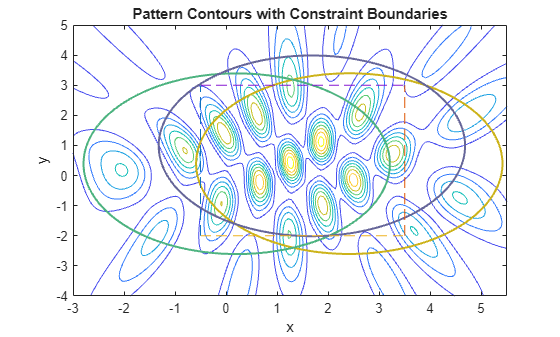### Setting Up and Solving the Problem with a Local Solver

Given the nonlinear constraints, we need a constrained nonlinear solver, namely, `fmincon`.

Let's set up a problem structure describing our optimization problem. We want to maximize the intensity function, so we negate the values returned form `waveIntensity`. Let's choose an arbitrary start point that happens to be near the feasible region.

For this small problem, we'll use `fmincon`'s SQP algorithm.

```% Pass additional parameters via an anonymous function: apertureConstraint_x = @(x) apertureConstraint(x,xcoords,ycoords); % Set up fmincon's options x0 = [3 -1]; opts = optimoptions('fmincon','Algorithm','sqp'); problem = createOptimProblem('fmincon','objective', ... @(x) -waveIntensity_x(x),'x0',x0,'lb',lb,'ub',ub, ... 'nonlcon',apertureConstraint_x,'options',opts); % Call fmincon [xlocal,fvallocal] = fmincon(problem) ```
```Local minimum found that satisfies the constraints. Optimization completed because the objective function is non-decreasing in feasible directions, to within the default value of the optimality tolerance, and constraints are satisfied to within the default value of the constraint tolerance. xlocal = -0.5000 0.4945 fvallocal = -1.4438 ```

Now, let's see how we did by showing the result of `fmincon` in our contour plot. Notice that `fmincon` did not reach the global maximum, which is also annotated on the plot. Note that we'll only plot the bound that was active at the solution.

```[~,maxIdx] = max(Z(:)); xmax = [X(maxIdx),Y(maxIdx)] figure contour(X,Y,Z) hold on % Show bounds line([lb(1) lb(1)],[lb(2) ub(2)],'LineStyle','--') % Create textarrow showing the location of xlocal annotation('textarrow',[0.25 0.21],[0.86 0.60],'TextEdgeColor',[0 0 0],... 'TextBackgroundColor',[1 1 1],'FontSize',11,'String',{'Single Run Result'}); % Create textarrow showing the location of xglobal annotation('textarrow',[0.44 0.50],[0.63 0.58],'TextEdgeColor',[0 0 0],... 'TextBackgroundColor',[1 1 1],'FontSize',12,'String',{'Global Max'}); axis([-1 3.75 -3 3]) ```
```xmax = 1.2500 0.4450 ```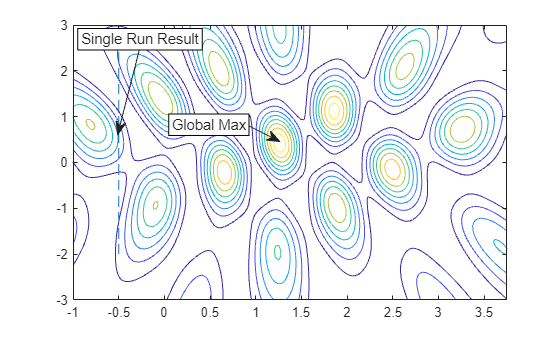### Using `GlobalSearch` and `MultiStart`

Given an arbitrary initial guess, `fmincon` gets stuck at a nearby local maximum. Global Optimization Toolbox solvers, particularly `GlobalSearch` and `MultiStart`, give us a better chance at finding the global maximum since they will try `fmincon` from multiple generated initial points (or our own custom points, if we choose).

Our problem has already been set up in the `problem` structure, so now we construct our solver objects and run them. The first output from `run` is the location of the best result found.

```% Construct a GlobalSearch object gs = GlobalSearch; % Construct a MultiStart object based on our GlobalSearch attributes ms = MultiStart; rng(4,'twister') % for reproducibility % Run GlobalSearch tic; [xgs,~,~,~,solsgs] = run(gs,problem); toc xgs % Run MultiStart with 15 randomly generated points tic; [xms,~,~,~,solsms] = run(ms,problem,15); toc xms ```
```GlobalSearch stopped because it analyzed all the trial points. All 14 local solver runs converged with a positive local solver exit flag. Elapsed time is 0.624779 seconds. xgs = 1.2592 0.4284 MultiStart completed the runs from all start points. All 15 local solver runs converged with a positive local solver exit flag. Elapsed time is 0.341500 seconds. xms = 1.2592 0.4284 ```

### Examining Results

Let's examine the results that both solvers have returned. An important thing to note is that the results will vary based on the random start points created for each solver. Another run through this example may give different results. The coordinates of the best results `xgs` and `xms` printed to the command line. We'll show unique results returned by `GlobalSearch` and `MultiStart` and highlight the best results from each solver, in terms of proximity to the global solution.

The fifth output of each solver is a vector containing distinct minima (or maxima, in this case) found. We'll plot the (x,y) pairs of the results, `solsgs` and `solsms`, against our contour plot we used before.

```% Plot GlobalSearch results using the '*' marker xGS = cell2mat({solsgs(:).X}'); scatter(xGS(:,1),xGS(:,2),'*','MarkerEdgeColor',[0 0 1],'LineWidth',1.25) % Plot MultiStart results using a circle marker xMS = cell2mat({solsms(:).X}'); scatter(xMS(:,1),xMS(:,2),'o','MarkerEdgeColor',[0 0 0],'LineWidth',1.25) legend('Intensity','Bound','GlobalSearch','MultiStart','Location','best') title('GlobalSearch and MultiStart Results') ```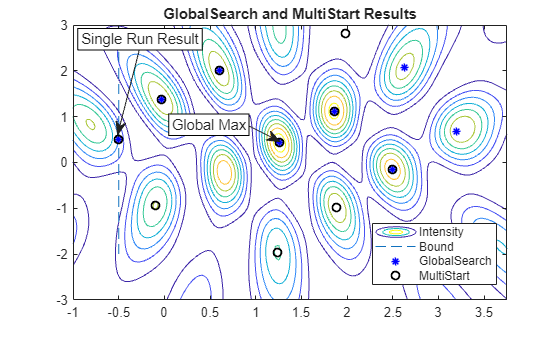### Relaxing the Bounds

With the tight bounds on the problem, both `GlobalSearch` and `MultiStart` were able to locate the global maximum in this run.

Finding tight bounds can be difficult to do in practice, when not much is known about the objective function or constraints. In general though, we may be able to guess a reasonable region in which we would like to restrict the set of start points. For illustration purposes, let's relax our bounds to define a larger area in which to generate start points and re-try the solvers.

```% Relax the bounds to spread out the start points problem.lb = -5*ones(2,1); problem.ub = 5*ones(2,1); % Run GlobalSearch tic; [xgs,~,~,~,solsgs] = run(gs,problem); toc xgs % Run MultiStart with 15 randomly generated points tic; [xms,~,~,~,solsms] = run(ms,problem,15); toc xms ```
```GlobalSearch stopped because it analyzed all the trial points. All 4 local solver runs converged with a positive local solver exit flag. Elapsed time is 0.482697 seconds. xgs = 0.6571 -0.2096 MultiStart completed the runs from all start points. All 15 local solver runs converged with a positive local solver exit flag. Elapsed time is 0.432552 seconds. xms = 2.4947 -0.1439 ```
```% Show the contours figure contour(X,Y,Z) hold on % Create textarrow showing the location of xglobal annotation('textarrow',[0.44 0.50],[0.63 0.58],'TextEdgeColor',[0 0 0],... 'TextBackgroundColor',[1 1 1],'FontSize',12,'String',{'Global Max'}); axis([-1 3.75 -3 3]) % Plot GlobalSearch results using the '*' marker xGS = cell2mat({solsgs(:).X}'); scatter(xGS(:,1),xGS(:,2),'*','MarkerEdgeColor',[0 0 1],'LineWidth',1.25) % Plot MultiStart results using a circle marker xMS = cell2mat({solsms(:).X}'); scatter(xMS(:,1),xMS(:,2),'o','MarkerEdgeColor',[0 0 0],'LineWidth',1.25) % Highlight the best results from each: % GlobalSearch result in red, MultiStart result in blue plot(xgs(1),xgs(2),'sb','MarkerSize',12,'MarkerFaceColor',[1 0 0]) plot(xms(1),xms(2),'sb','MarkerSize',12,'MarkerFaceColor',[0 0 1]) legend('Intensity','GlobalSearch','MultiStart','Best GS','Best MS','Location','best') title('GlobalSearch and MultiStart Results with Relaxed Bounds') ```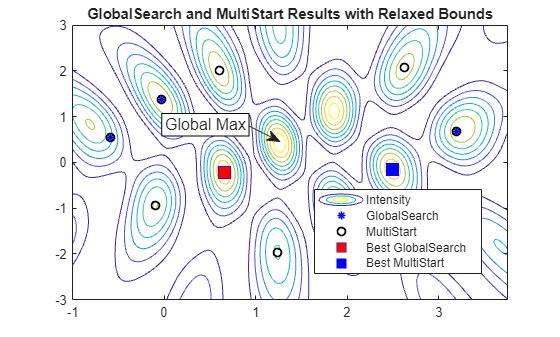The best result from `GlobalSearch` is shown by the red square and the best result from `MultiStart` is shown by the blue square.

### Tuning `GlobalSearch` Parameters

Notice that in this run, given the larger area defined by the bounds, neither solver was able to identify the point of maximum intensity. We could try to overcome this in a couple of ways. First, we examine `GlobalSearch`.

Notice that `GlobalSearch` only ran `fmincon` a few times. To increase the chance of finding the global maximum, we would like to run more points. To restrict the start point set to the candidates most likely to find the global maximum, we'll instruct each solver to ignore start points that do not satisfy constraints by setting the `StartPointsToRun` property to `bounds-ineqs`. Additionally, we will set the `MaxWaitCycle` and `BasinRadiusFactor` properties so that `GlobalSearch` will be able to identify the narrow peaks quickly. Reducing `MaxWaitCycle` causes `GlobalSearch` to decrease the basin of attraction radius by the `BasinRadiusFactor` more often than with the default setting.

```% Increase the total candidate points, but filter out the infeasible ones gs = GlobalSearch(gs,'StartPointsToRun','bounds-ineqs', ... 'MaxWaitCycle',3,'BasinRadiusFactor',0.3); % Run GlobalSearch tic; xgs = run(gs,problem); toc xgs ```
```GlobalSearch stopped because it analyzed all the trial points. All 10 local solver runs converged with a positive local solver exit flag. Elapsed time is 0.622113 seconds. xgs = 1.2592 0.4284 ```

### Utilizing `MultiStart`'s Parallel Capabilities

A brute force way to improve our chances of finding the global maximum is to simply try more start points. Again, this may not be practical in all situations. In our case, we've only tried a small set so far and the run time was not terribly long. So, it's reasonable to try more start points. To speed the computation we'll run `MultiStart` in parallel if Parallel Computing Toolbox™ is available.

```% Set the UseParallel property of MultiStart ms = MultiStart(ms,'UseParallel',true); try demoOpenedPool = false; % Create a parallel pool if one does not already exist % (requires Parallel Computing Toolbox) if max(size(gcp)) == 0 % if no pool parpool demoOpenedPool = true; end catch ME warning(message('globaloptim:globaloptimdemos:opticalInterferenceDemo:noPCT')); end % Run the solver tic; xms = run(ms,problem,100); toc xms if demoOpenedPool % Make sure to delete the pool if one was created in this example delete(gcp) % delete the pool end ```
```MultiStart completed the runs from all start points. All 100 local solver runs converged with a positive local solver exit flag. Elapsed time is 2.641997 seconds. xms = 1.2592 0.4284 ```

### Objective and Nonlinear Constraints

Here we list the functions that define the optimization problem:

```function p = waveIntensity(x,amp,phase,relFreqConst,numSources,origins) % WaveIntensity Intensity function for opticalInterferenceDemo. % Copyright 2009 The MathWorks, Inc. d = distanceFromSource(x,numSources,origins); ampVec = [sum(amp./d .* cos(phase - d*relFreqConst)); sum(amp./d .* sin(phase - d*relFreqConst))]; % Intensity is ||AmpVec||^2 p = ampVec'*ampVec; ```
```function [c,ceq] = apertureConstraint(x,xcoords,ycoords) % apertureConstraint Aperture constraint function for opticalInterferenceDemo. % Copyright 2009 The MathWorks, Inc. ceq = []; c = (x(1) - xcoords).^2 + (x(2) - ycoords).^2 - 9; ```
```function d = distanceFromSource(v,numSources,origins) % distanceFromSource Distance function for opticalInterferenceDemo. % Copyright 2009 The MathWorks, Inc. d = zeros(numSources,1); for k = 1:numSources d(k) = norm(origins(k,:) - [v 0]); end ```